Harmonic Analysis of Roundness Data

What is Harmonic Analysis?

“Harmonic Analysis” sounds impressive doesn’t it?  Don’t let it scare you – it’s a fancy mathematical way of describing things that move (or shake) back and forth. If a pen was oval it would bounce twice per revolution if you rolled it on your desktop.  That’s a 2nd order harmonic.  (2nd order means 2x per revolution).  Many wood pencils are 6 sided.  Thus, they have a strong waveform with bumps occurring six times per revolution.  We can also say this a different way:  the pencil has a strong amplitude at six “undulations per revolution” (UPR).  An “undulation” can be thought of as a sine wave or a “cycle”.

With those sentences, we just did “harmonic analysis” … using words.  We can also do this with math and software.

Harmonic analysis looks at your measured data and tells you “how many times” the bumps occur in each revolution.  In fact, harmonic analysis actually looks at every frequency (every UPR) and reports how tall the bumps are for each of them.  That’s a powerful analysis.

Digging in a litter deeper…

This ugly shape in the center of this figure is made up of 4 different “pure shapes” that are added together.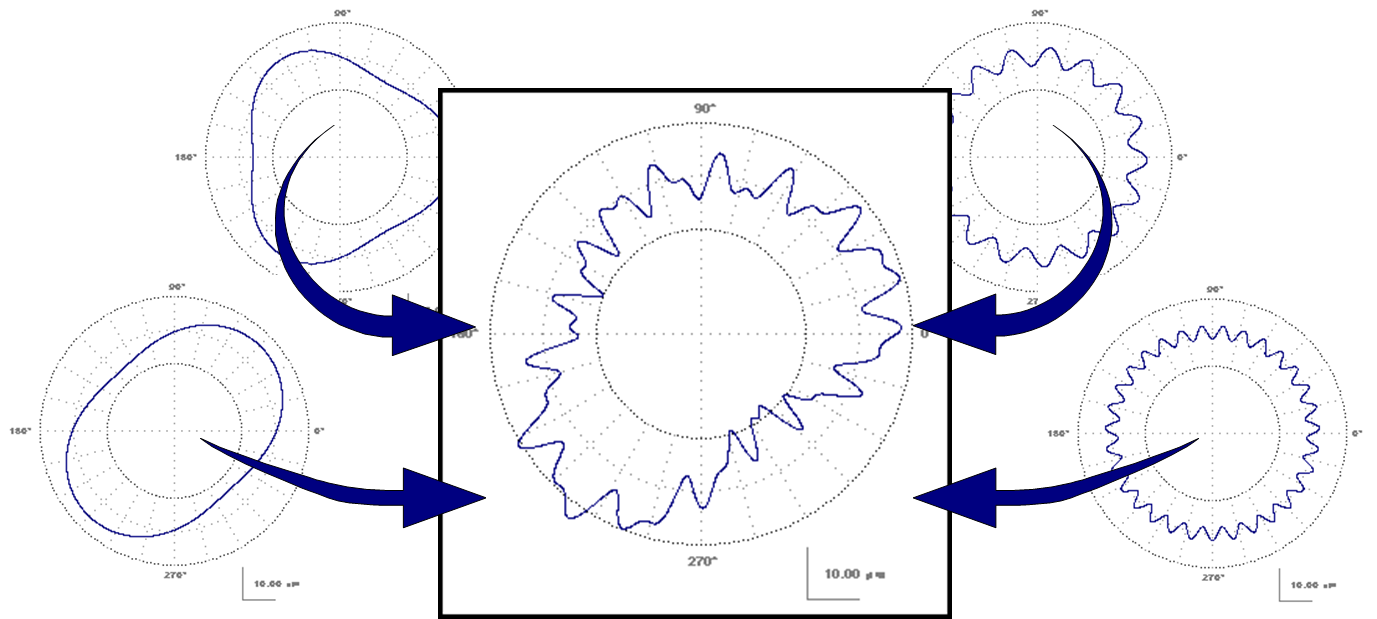Each of the four shapes around the edges represent a “harmonic”.  A harmonic is sine wave that occurs a certain number of times per revolution.  This center shape is made up of four different harmonics.  In this example, the harmonics are 2nd order (lower left), 3rd order (upper left), 17th order (upper right) and 31st order (lower right).

Harmonic analysis works in the opposite direction.  Instead of combining simple shapes to make a complicated shape – harmonic analysis starts with the complicate shape and breaks it into simple sine waves.  Typically, a graph or table is provided to tell you exactly how much of each harmonic is present in the data.  In this example we see a bar graph.  The taller bars indicate that there is “more” of that specific harmonic.  For our current example with 4 harmonics, the bar graph would look like this: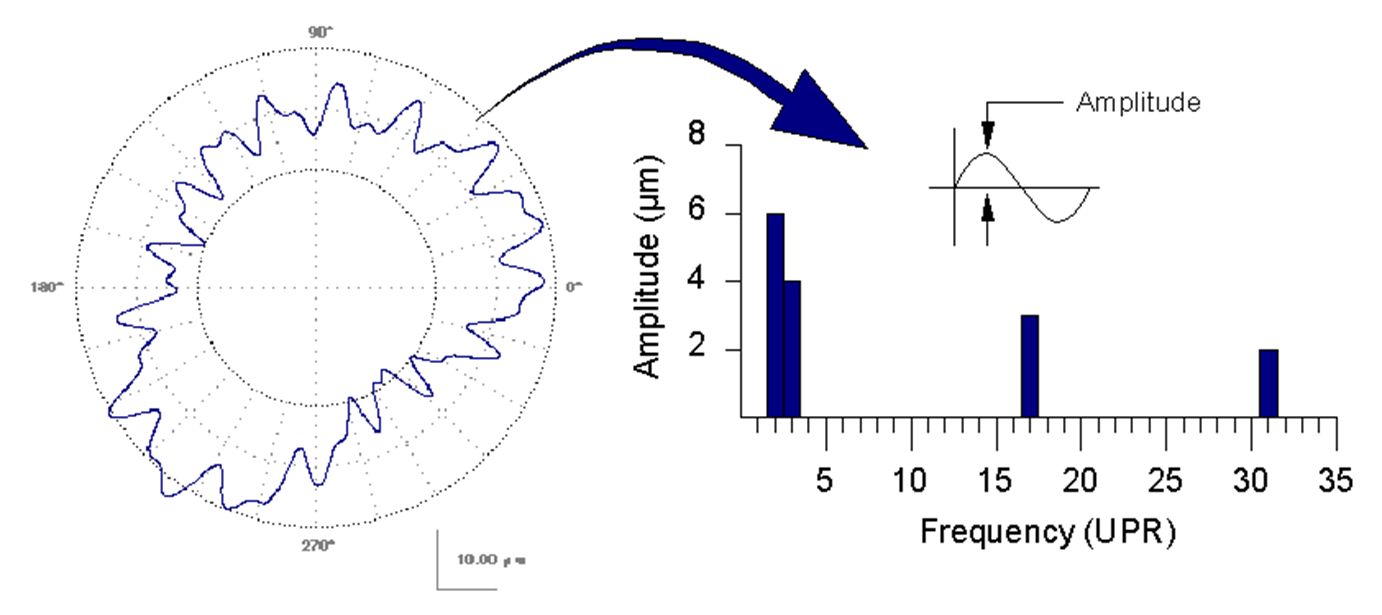The 4 bars indicate that there is significant “amplitude” (or “height”) at these frequencies.   On thing that this graph often leaves out is the “phase” of the harmonic.  For example, the 2nd order (oval) component is currently oriented with the first peak at 45°.  The phase of each harmonic tells us where the first peak occurs.

One thing that needs to be managed with harmonic analysis is what you mean by the word “amplitude”.  Some people specify “mean-to-peak” amplitude while others use “peak-to-valley” amplitude.  It is important to get this term right – especially if you are setting a tolerance on peak-to-valley and your supplier is measuring mean-to-peak!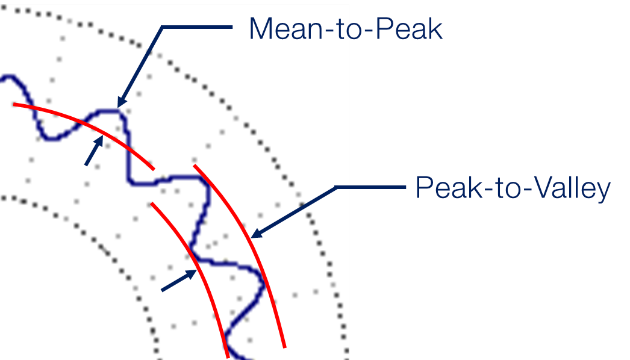What can we do with this?

One thing that we can do with harmonics is to put tolerances on them.  This can be done with a table of harmonic limits.  Or in other cases an equation that sets a limit “curve”.  Below is an example from OmniRound with the harmonic limit curve being set by an equation.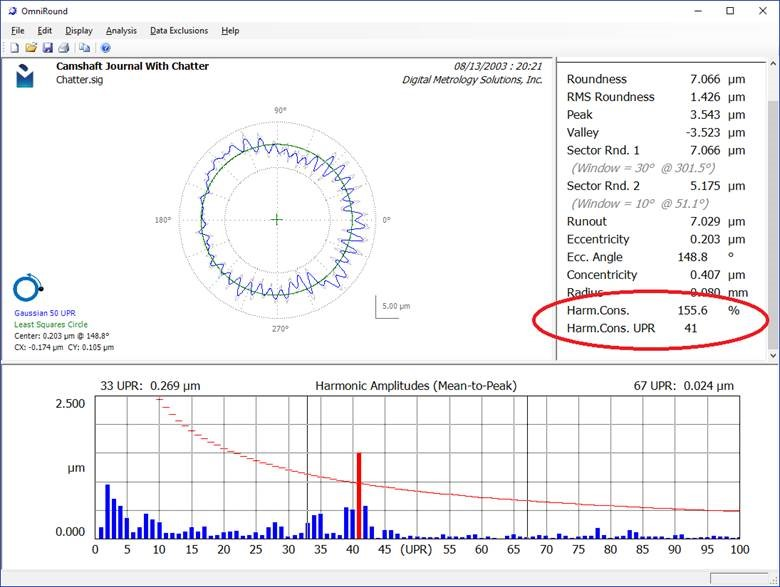OmniRound highlights the bars that exceed the tolerance limit.  Furthermore, OmniRound detects the worst harmonic (the one that uses the most of its tolerance) and reports the amount of tolerance it consumes.  This is shown has the Harmonic Consumption (Harm.Cons.) parameter.  In the above example, the chatter limit is exceeded at 41 UPR.  This is shown on the graph and in the “Harm.Cons. UPR” parameter.

The Harm.Cons. parameter is a powerful way to combine harmonic analysis across hundreds of UPR and turn it into a single number.  For a surface to be acceptable, all harmonics must lie below the tolerance curve.  Thus, the Harm.Cons parameter must be below 100%.

Conclusion

Harmonic analysis is a powerful tool for understanding your roundness data.  Check out Digital Metrology’s “OmniRound” software and get started with your own harmonic analysis for your data sets!

The measurement of roundness is often debated.

Many of these debates are centered around the tip radius and filtering used in the measurement.  This debate is further complicated by the fact that the typical roundness graph distorts reality.

Here is the same roundness data plotted at varying magnifications…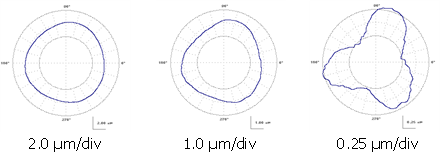When we look at a roundness graph for an outside diameter measurement we see locally concave areas.  Often these areas look rather sharp.  This is due to the polar plot scaling and the magnification of “errors” rather than the magnification of the “size” .

The rightmost plot appears as though there are large concave areas on this component.  However, this data is from a precision gage ball.  The actual workpiece has no concave areas as the polar plot seems to indicate.  In fact the actual surface is completely convex and, given the magnitude of the roundness errors, a flat follower would have no problem measuring the roundness of this surface.

One other problem arises from a polar plot and it’s “vanishing point” at the center of the plot.  Since negative values all approach the center of the plot, they tend to “compress”.  Positive values tend to “fan out”.  This gives the perception that inward features are much sharper than outward features: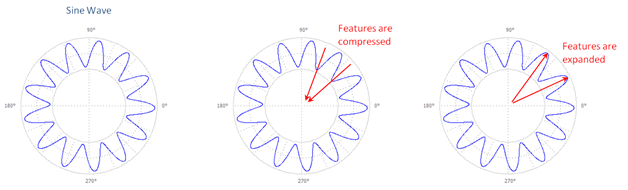When we increase the scale, we see a large (apparent) difference between peak and valley curvatures: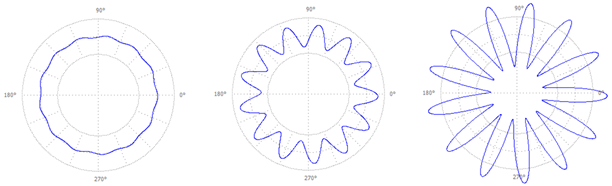On the right most graph (above) we get the perception that the valley bottom becomes a sharp point.  However, this is not the case with the actual data.

So let’s look at sine waves and think about the concept of “radius of curvature”…

Linear Sine Waves and their Radii

The radius of curvature of a sine wave (either peak or valley) is given by: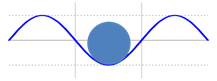Where,

RSINE        the smallest radius of the sine wave (for example at the valley).  See Figure A4.

l             the wavelength of the sine wave

A             the amplitude of the sine wave

Since we are dealing with circular parts, the wavelength, l, is based on: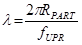Where,

RPART       the radius of the part being measured

fUPR         the frequency of interest expressed in undulations per revolution (UPR)

which ultimately gives the sine wave radius on a circular part as: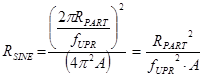What about Inside and Outside Diameters?

If we are measuring an outside diameter, we can use a larger tip radius to see all of the features of a given sine wave.  If we are measuring an inside diameter we will need a smaller radius in order to see the same features:  This figure shows a sine wave wrapped around a circle.  In doing so, the spacing between the peaks of the sine wave are effectively “widened” as compared to a sine wave on a straight reference line: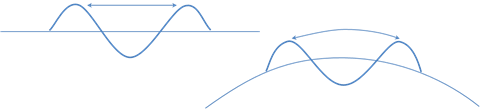Ideally, we would like to combine the radius of curvature of the part with the radius of curvature of the sine wave.  Unfortunately, radius values do not mathematically combine, but curvature values do combine.  Mathematically, curvature is the inverse of the radius of curvature:For an outside diameter, the largest allowable tip has a curvature of: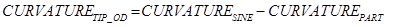For an inside diameter, the curvatures add: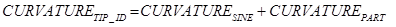Thus for an Outside Diameter: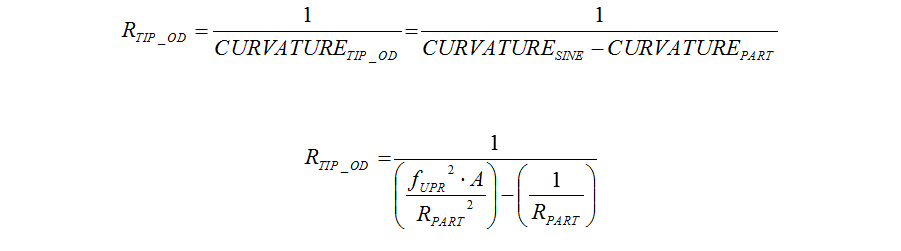Outside diameter considerations: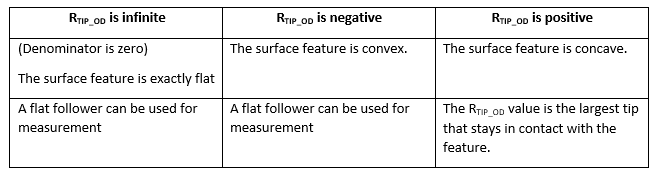Similarly, for an Inside Diameter:Where:

A             the amplitude of the sine wave

RPART       the radius of the part being measured

fUPR         the frequency of interest expressed in undulations per revolution (UPR)

The above defined tip radius will actually touch the bottom of the sine wave, however it will only occur at a discrete point and will cause a “cusp” or “sharp corner”.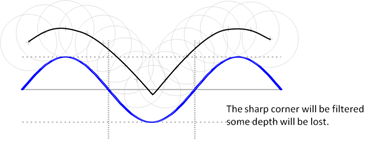Since the cusp is very sharp, it will be filtered and some of the depth will be lost.  Thus, a smaller tip should be used in order to gain additional confidence.  A reduction of 25% may be adequate as a safety margin.

A 10 mm inside diameter has a roundness limit of 0.005 mm with a 50 UPR filter cutoff: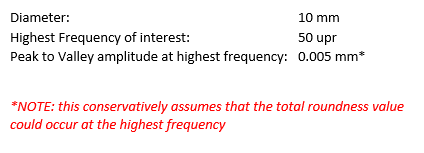Using the inside diameter equation: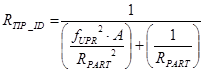… and assuming that all of the peak-to-valley roundness error occurs at the highest frequency we have: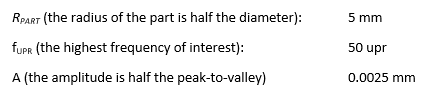Inserting values: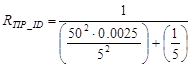Which gives a max tip radius of: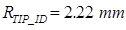A 25% safety factor (multiply by 0.75) gives: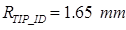Conclusion

Roundness may continue to be debated.  But this discussion shows that the relationship between surface waveforms and the tip radius can be understood.

For more help understanding your toughest metrology challenges (and help with settling your debates) contact Digital Metrology today!

Filtering for Roughness and Waviness

Check out this new animation!  This is based on some of Digital Metrology’s training materials and shows how the roughness and waviness profiles change with a changing filter cutoff.

The profile is from a milled surface with a 2.5 mm feed rate.

• The top left graph shows the primary & waviness profiles.
• The top right shows the wavelength content and the filter transmission curves
• The bottom graphs show roughness (left) and waviness (right)

Enjoy!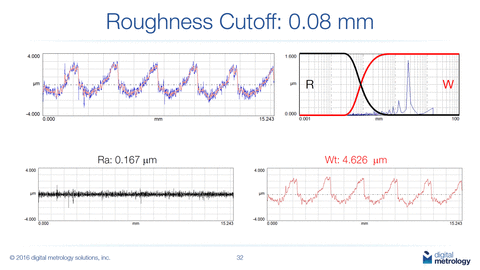To find out more about your surfaces, or to schedule a training session contact Digital Metrology today!